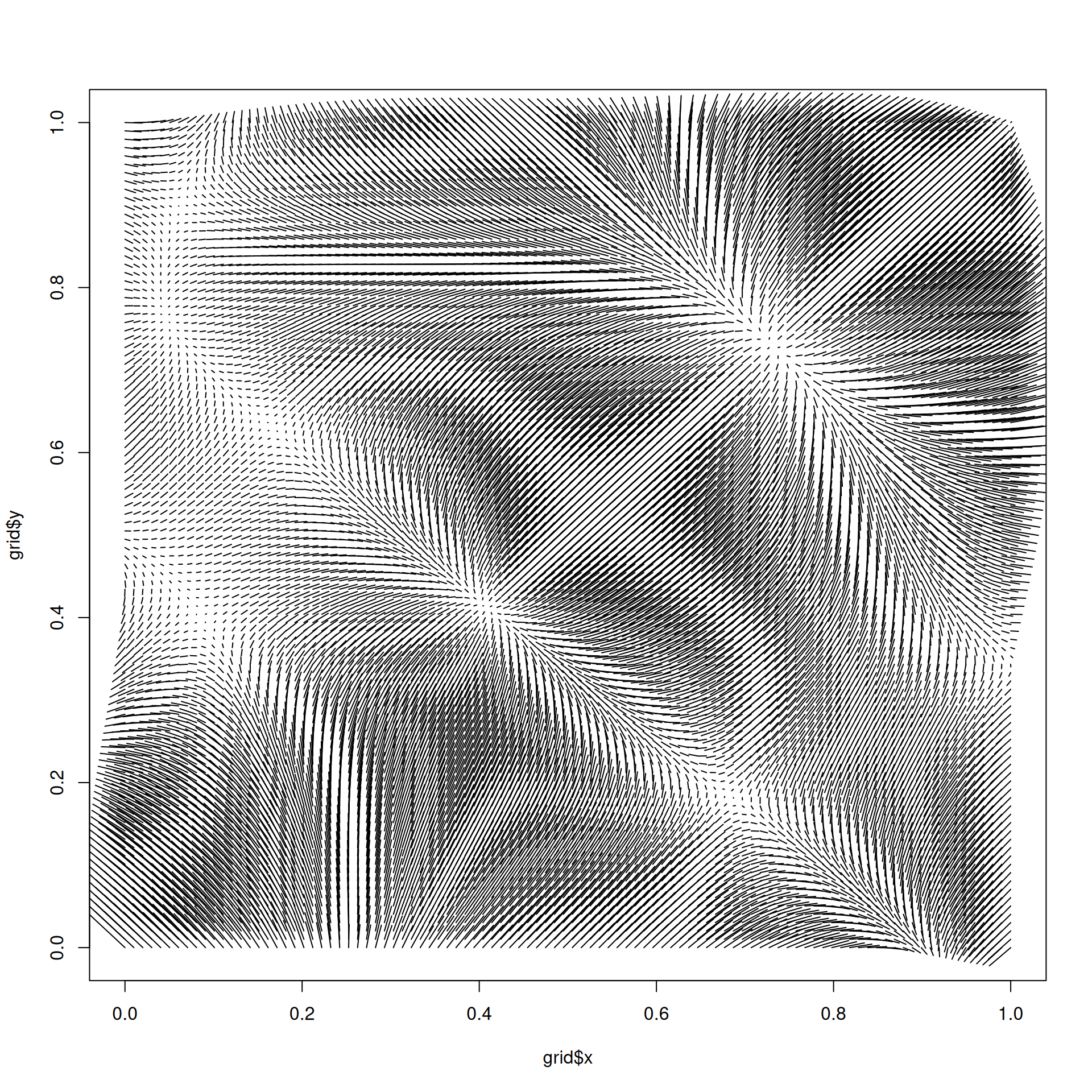The gradient of a scalar field such as those generated by the different noise algorithms in ambient is a vector field encoding the direction to move to get the strongest increase in value. The vectors generated have the properties of being perpendicular on the contour line drawn through that point. Take note that the returned vector field flows upwards, i.e. points toward the steepest ascend, rather than what is normally expected in a gravitational governed world.

gradient_noise(
generator,
x,
y,
z = NULL,
t = NULL,
...,
seed = NULL,
delta = NULL
)

## Arguments

generator The noise generating function, such as gen_simplex, or fracture() The coordinates to generate the gradient for as unquoted expressions Further arguments to generator A seed for the generator. The offset to use for the partial derivative of the generator. If NULL, it will be set as 1e-4 of the largest range of the dimensions.

Other derived values: curl_noise()
grid <- long_grid(seq(0, 1, l = 100), seq(0, 1, l = 100))
grid$gradient <- gradient_noise(gen_simplex, x = grid$x, y = grid$y) plot(grid$x, grid$y, type = 'n') segments(grid$x, grid$y, grid$x + grid$gradient$x / 100, grid$y + grid$gradient\$y / 100)